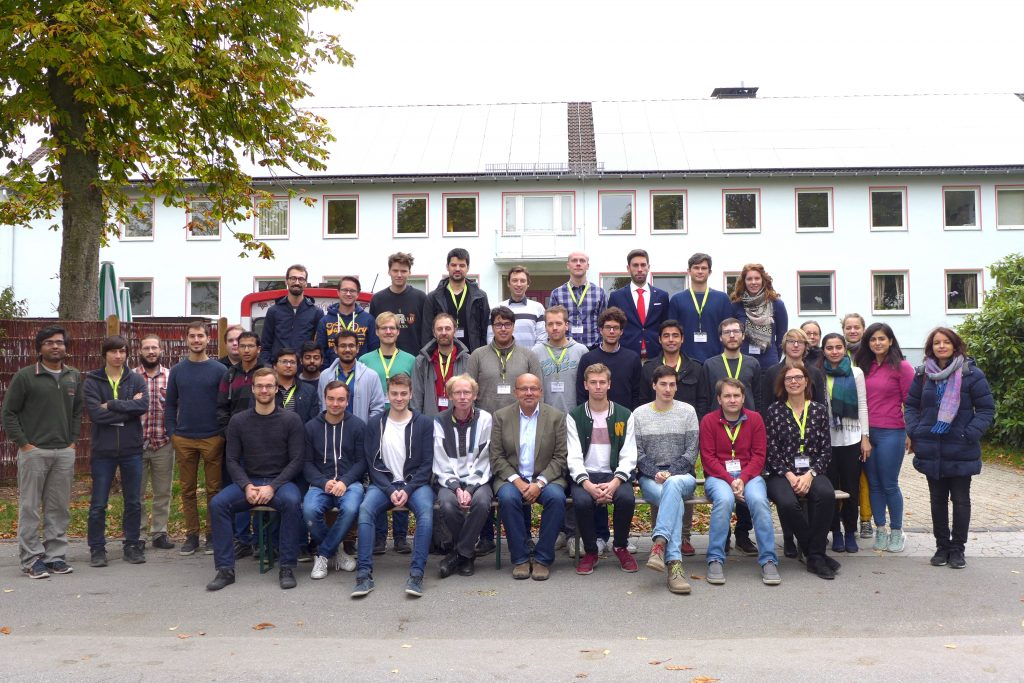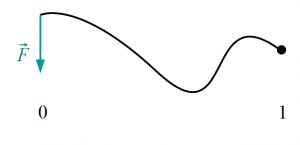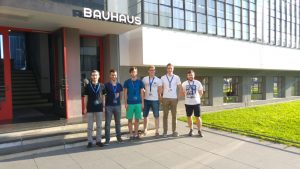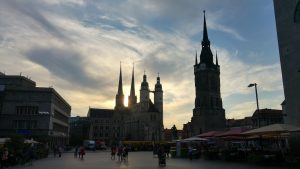Control Theory – is it an exciting topic? Impressions from the Summer School on Control of Evolution Equations @ Wuppertal

From September 30 to October 4, 2018 the Summer School on Control of Evolution Equations took place in Wuppertal and I appreciated it very much to be one of its participants. The program was divided into two interesting topics. Both lecture sessions shared an open and very enjoyable atmosphere due to the enthusiasm of the lecturers, Hans Zwart (Twente) and Marius Tucsnak (Bordeaux). My participation in the summer school lead to this blog article about control theory.Participants of the Summer School on Control of Evolution Equations @ Wuppertal

After this short introduction, some of you might have noticed that I have not answered the question in the title – a summer school with great lectures does not necessarily mean that the topic itself  is interesting or even exciting. Maybe you even want to add the following questions:

What are the main issues and goals of control theory?
How is it related to the CRC on wave phenomena?

As usual, the best way to motivate the concepts of control theory and try to answer all these questions is to use an example, which I encountered in the lectures of Marius Tucsnak and Hans Zwart. For the sake of simplicity and evidence, I do only select the single example of a one-dimensional vibrating string. Nevertheless, this summer school taught me that there are many other examples having interesting connections to various parts within our CRC. If you are further interested in the example, it can be found in chapters 6.2 and 11.2 of the book Observation and Control for Operator Semigroups by M. Tucsnak and G. Weiss (Birkhäuser 2009) as well as chapter 9.2 of the book Linear Port-Hamiltonian Systems on Infinite-dimensional Spaces by B. Jacob and H. Zwart (Birkhäuser 2012). Note furthermore that control theory is a wide subject, so I will only motivate the three concepts of observability, controllability and stabilizability.

Consider a vibrating elastic string (of your favorite length, say 1) that is fixed at both ends, which models for instance the string of a violin. First, there are no external forces acting on it (no gravity, damping, etc.). We call the vibrating string our system and the amplitude of its oscillations behaves according to a one-dimensional wave equation. Imagine now, that we do not know anything about the state of our system, i.e., its initial and current shape is not visible. Consequently, our system has become a black box and we cannot deduce anything about it.
So, we assume that we can measure an output of our system, say the slope at its left end (i.e., the spatial derivative at this point). One natural question concerns (exact) observability: can we deduce the initial (and thus the current) state of our system by means of our observations? The answer is yes, but we must observe our system on time scales larger than 2 which is also called the minimal observation time.

However, observing is not sufficient for most applications – acting (or controlling) is better. Thus, we follow up the path of control theory and get to the aspect of controllability. The goal of (exact) controllability is to move our system to a given state within a given time. The port over which we can manipulate the system is called input. That definition sounds quite abstract, so how is controllability related to our string? Let us unloose our string at the left end, so that it can move freely here. We now manipulate our system by means of a force that acts on the loose left end of the string and is caused by a damper or an engine. In this case, our system is exactly controllable for every time larger than 2 and this means the following. Suppose, we have a given initial shape $$w_{\text{start}}$$, an initial velocity $$\partial_t w_{\text{start}}$$ and a certain desired final shape $$w_{\text{final}}$$ as well as a final velocity $$\partial_t w_{\text{final}}$$ that our system should attain at a time $$T$$ larger than 2. Then we can find a time-dependent force that acts on the loose left end of our string during the time $$[0,T]$$ and drives its state from $$(w_{\text{start}},\partial_t w_{\text{start}})$$ to the desired one $$(w_{\text{final}},\partial_t w_{\text{final}})$$ at time $$T$$.A vibrating elastic string, fixed at its right end and free at its left. In our model, we influence our string via a force acting on the left end.

Our system with a damping force acting on the left end of our string is also a suitable instance of the concept of stabilization. Can we design a damper in such a way that the oscillations of our string decay in a uniform way, say exponentially, on large time intervals? Let us assume that we can observe also the vertical velocity of our string at the left end. Now we construct a damper that induces a force being negatively proportional to the string’s velocity at its left end.  In this way we have designed a closed loop system, i.e., we have constructed a control (our input force) based on the knowledge of a given output (the observable velocity at the left end). Returning to our goal of stabilization: the oscillations of our closed loop system decay indeed in a uniform exponential way. Of course, there are many other ways of designing controls based on certain outputs and one can also ask for cost-optimal controls and the numerical approximation of controls.

During summer school, we considered many other examples of control theory for wave equations such as coupled strings, vibrating plates and other higher dimensional wave equations on various domains. Most fascinating for me is the interplay of abstract functional analysis with complex analysis having in mind real applications such as the design of machines by engineers – think of the manufacture of integrated circuits for computer chips requiring machines that are moving fast with extreme precision. In this way we have returned to the question from the title and I hope, I could convince you that control theory is indeed an exciting topic and worth to deal with.

NUMDIFF-15 conference in Halle (Saale)f.l.t.r. Konstantin Zerulla, Jan Leibold, Jan Philip Freese, Patrick Krämer, Bernhard Maier, Benjamin Dörich

From the 3rd to the 7th of September six researchers of our CRC visited the NUMDIFF-15 Conference which took place in Halle (Saale), Germany. The conference, hosted by the Martin-Luther-University Halle-Wittenberg, addressed all aspects of numerical simulation of differential equations. This time the emphasis was put on differential-algebraic equations (DAE) and stochastic differential equations (SDE).

We are proud to have represented our CRC  by contributing talks about the analytical and numerical treatment of wave phenomena to the conference. The scientific program contained many inspiring talks even though not all of them matched the research topics of our CRC.Market Square of Halle

The time we did not spend on the conference site we used to explore the lovely city center of Halle, Handel’s native town. On Wednesday we took part in the excursion to the UNESCO World Cultural Heritage Bauhaus Dessau. In a guided tour we did not only learn a lot about the worldwide influence of Bauhaus in architecture and design but also about its history which is closely related to German history.

We are looking forward to contribute to the NUMDIFF-16 taking place in three years.